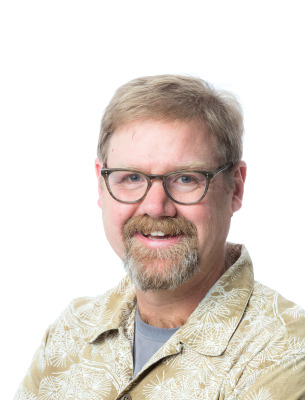# Peter Staab

PROFESSOR
Mathematics School of Health and Natural SciencesOffice: Edgerly Hall 310A
Office Hours
Fall 2023

Monday 12:30pm - 1:45pm
Thursday 11:00am - 12:15pm

## Courses Taught

Finite Mathematics (MATH 1200)
Precalculus (MATH 1300)
Informal Math Modeling (MATH 1600)
Technical Calculus (MATH 2100)
Calculus I (MATH 2300)
Calculus II (MATH 2400)
Methods of Applied Mathematics (MATH 3500)
Ordinary Differential Equations (MATH 3550)
Math Seminar (MATH 3900)
Operations Research (MATH 4400)
Mathematical Modeling (MATH 4450)
Numerical Analysis (MATH 4500)

## Background

Ph.D., University of Colorado Boulder, 1998

Image Processing
Fluid Mechanics
Dynamical Systems
Numerical Analysis
Partial Differential Equations
Mathematical Pedagogy

Ph.D., University of Colorado Boulder, 1998

Ph.D., University of Colorado Boulder, 1998